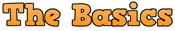# Lottery MathLottery Math is slightly different than the regular math operations. This article will provide a high-level overview of the Lottery Math used by most of the Lottery Players.

The basic rule followed in the Lottery Math is that when adding or subtracting numbers using lottery math, we do not carry over or borrow from.

Example: 4 + 8 = 2 and not 12; 1 – 3 = 8 and not -2

Few other math operations commonly used in Lottery workouts are following:

SUMS: To get the SUM of a number, we simply add all the digits together. For example, if the number drawn was 189, the SUM would be 1 + 8 + 9 =18

BASE SUM: The BASE Sum is simply the last digit of the drawn number. If there is only one digit in the SUM, then BASE SUM is same as the SUM.

Examples:

• If SUM = 8, then BASE SUM = 8
• If SUM = 16, then BASE SUM = 6
• If SUM = 22, then BASE SUM = 2

ROOT SUM: In order to find the ROOT SUM of the drawn number, continue to add the SUM of the number until a single digit is returned. That single digit is called the ROOT SUM of the number.

Examples:

• Number Drawn: 225, the SUM is 2 + 2 + 5 = 9; Since 9 is a single digit, 9 is also the ROOT SUM.
• Number Drawn: 446, The SUM is 4 + 4 + 6 = 14 SUM, then add 1 + 4 = 5 (single digit), which is the ROOT SUM.
• Number Drawn 757, the SUM is 7 + 5 + 7 = 19 SUM, then add 1 + 9 = 10 (double digit), then 1 + 0 = 1 (single digit), which is the ROOT SUM.

Therefore, in lottery math, if the number drawn is 277, we should also refer the number as following:

1. It is a Double Number.
2. LE, HO, HO Number. (LO: Low Odd, HO: High Odd)
3. It has a SUM of 16 (2 + 7 + 7 = 16)
4. It has a BASE SUM of 6
5. It has a ROOT SUM of 6 (Sum = 16 = 1 + 6 = Root Sum 7)

DATE SUM: There are multiple ways how lottery players calculate DATE SUM. All the methods are equally good and it is purely up-to the individual and back testing process, to use one over the another.

DATE Sum Version 1:  Add Month, Day and Year part of the Date together and derive the ROOT SUM from the Date.

Example:  05 April, 2019: – 04 (Month) + 05 (Day) + 2019 = SUM 21 = ROOT SUM 2 + 1 = 3

DATE Sum Version 2: Add Month and Day part only and derive the ROOT SUM.

Example:  05 April, 2019: – 04 (April) + 05 (Day) = SUM 9 = ROOT SUM 9

Article Rating
Subscribe
Notify of
Inline Feedbacksacmorwin
3 years ago

Just so you know….Your second example where number drawn is 446 does not add up to 16 but 14; 4+4+6=14 aka 1+4=5 making 5 the root sum according to your lottery mathDominic Ward
3 years ago

My only question is after you find the Date,Root, and Hit Sum how can you narrow down your search even more to find at least 5-10 good digits to use for the draw not even counting the mirror numbers that most states add in?Witty
3 years ago

You will have to look for the history and find out the overdue Sums, Root Sums etc. That will help you narrow down the results. Please keep in mind, even though the lottery is game of chance, it does follow some of the statistical and probability rules over the period of time. Therefore attributes which are super overdue are always the hot ones to come soon. Such additional filters will help you to narrow down the number combinations significantly along with workouts and other pets. Hope this helps.Gwen Mathew
3 years ago

Hello Everyone
Any luck! No luck for me😰denis putt
3 years ago

Hello Gwen…..Not winning???? Try this. Look at my post with the pairs. If it doesn’t double or triple, you already have two(2) of the winning digits with one of my pairs…..then, Look at your states prediction. Find one of my pairs the touches and/or uses the highlighted numbers. Play those few numbers and you should hit. thats what Im doing and winning…Witty
3 years ago

This is what works to me Sum 10 and Root Sum 1. one of these usually comes every 3-4 draws in a state or at least once everyday across all states. Study your state, there will be few root sums which comes every 3-4 draw in your state also, stick to those and Win. Good Luck.Gwen Mathew
3 years ago